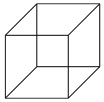Definition Of Cube

A Cube is a three-dimensional figure with six square faces.Example of Cube

A dice is in the form of a cube.

Solved Example on Cube

Ques: Find the total surface area of a cube of side 2 cm.

Choices:

A. 24 square centimeters
B. 12 square centimeters
C. 10 square centimeters
D. 2 square centimeters